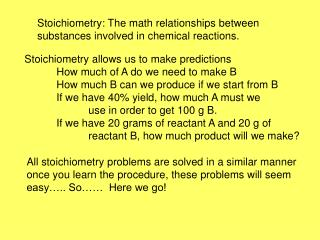DownloadDownload PresentationStoichiometry: The math relationships between substances involved in chemical reactions.

# Stoichiometry: The math relationships between substances involved in chemical reactions.

Télécharger la présentation## Stoichiometry: The math relationships between substances involved in chemical reactions.

- - - - - - - - - - - - - - - - - - - - - - - - - - - E N D - - - - - - - - - - - - - - - - - - - - - - - - - - -
##### Presentation Transcript

1. Stoichiometry: The math relationships between substances involved in chemical reactions. Stoichiometry allows us to make predictions How much of A do we need to make B How much B can we produce if we start from B If we have 40% yield, how much A must we use in order to get 100 g B. If we have 20 grams of reactant A and 20 g of reactant B, how much product will we make? All stoichiometry problems are solved in a similar manner once you learn the procedure, these problems will seem easy….. So…… Here we go!

2. 1. How many grams of NaAlO2 can be obtained from 4.46 g of aluminum chloride according to the following reaction? AlCl3 + 4 NaOH  NaAlO2 + 3 NaCl + 2 H2O ? g 4.46 g Al = 27.0 3Cl = 3(35.4) 133.2 g/mole gfw AlCl3 gfw NaAlO2 mole AlCl3 mole NaAlO2 Na = 23.0 Al = 27.0 2O = 32.0 82g/mole NaAlO2 mole ratio 82.0g NaAlO2 Mole NaAlO2 4.46 g AlCl3 Mole AlCl3 133.2 g AlCl3 1mole NaAlO2 1 mole AlCl3 = 2.74 g NaAlO2

3. 2. How many grams of hydrochloric acid are required to react completely with 61.8 g of calcium hydroxide? 2 HCl + Ca(OH)2 2 H2O + CaCl2 ? g 61.8 g H = 1.0 Cl = 35.4 36.4 g/mole HCl gfw HCl gfw Ca(OH)2 mole HCl mole Ca(OH)2 Ca = 40.1 2O = 32.0 2H = 2.0 74.0g/mole Ca(OH)2 mole ratio 36.4 g HCl mole HCl Mole Ca(OH)2 74.0 g Ca(OH)2 61.8 g Ca(OH)2 2mole HCl 1 mole Ca(OH)2 = 60.8 g HCl

4. 1. 3.How many grams of hydrogen are produced when 4.72 g of aluminum reacts with excess sulfuric acid? 2 Al + 3 H2SO4  3 H2 +Al2(SO4)3 Al = 27.0 g/mole ? g 4.72 g gfw Al gfw H2 mole Al mole H2 2 H = 2.00 g/mole mole ratio 2.00 g H2 mole H2 4.72 g Al mole Al 27.0 mole Al 3 mole H2 2mole Al = 0.524 g H2

5. 4. The action of carbon monoxide on iron(III) oxide can be represented by the equation Fe2O3 + CO  Fe + CO2. What is the minimum mass of carbon monoxide produced if 57.5 grams of iron were produced. (Hint) Make sure the equation is balanced before beginning. Fe2O3 + 3 CO  2 Fe + 3 CO2 ? g 57.5 g gfw Fe gfw CO C = 12.0 O = 16.0 28 g/mole CO moles CO moles Fe mole ratio mole Fe 55.8 g Fe 3 mole CO 2 mole Fe 28.0 g CO mole CO 57.5 g Fe

6. 6.Claude-Louis Berthollet first prepared ethyne (acetylene) by sparking carbon electrodes in hydrogen gas. 2C + H2 C2H2 How many grams of the carbon electrode will be consumed when 59.8 g of acetylene are produced? 2C + H2 C2H2 ?g 59.8 g ÷26 g/mole (59.8/26)mole C2H2 Answer: 55.2 g C Show how we got this answer!